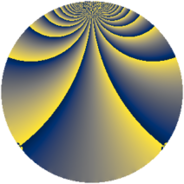# Properties

 Label 819.2.fyLevel $819$ Weight $2$ Character orbit 819.fy Rep. character $\chi_{819}(50,\cdot)$ Character field $\Q(\zeta_{12})$ Dimension $336$ Newform subspaces $1$ Sturm bound $224$ Trace bound $0$

# Related objects

## Defining parameters

 Level: $$N$$ $$=$$ $$819 = 3^{2} \cdot 7 \cdot 13$$ Weight: $$k$$ $$=$$ $$2$$ Character orbit: $$[\chi]$$ $$=$$ 819.fy (of order $$12$$ and degree $$4$$) Character conductor: $$\operatorname{cond}(\chi)$$ $$=$$ $$117$$ Character field: $$\Q(\zeta_{12})$$ Newform subspaces: $$1$$ Sturm bound: $$224$$ Trace bound: $$0$$

## Dimensions

The following table gives the dimensions of various subspaces of $$M_{2}(819, [\chi])$$.

Total New Old
Modular forms 464 336 128
Cusp forms 432 336 96
Eisenstein series 32 0 32

## Trace form

 $$336q - 12q^{6} + 36q^{8} + O(q^{10})$$ $$336q - 12q^{6} + 36q^{8} - 12q^{11} + 4q^{15} + 168q^{16} - 4q^{18} - 4q^{21} + 8q^{24} - 96q^{26} + 24q^{27} - 120q^{30} - 72q^{32} + 36q^{33} + 72q^{38} + 8q^{39} - 48q^{41} - 76q^{45} + 24q^{48} - 24q^{50} - 36q^{52} + 72q^{54} + 32q^{57} + 36q^{58} - 72q^{59} + 4q^{60} + 36q^{62} - 8q^{63} - 72q^{65} - 20q^{66} - 48q^{71} - 176q^{72} - 60q^{75} - 124q^{78} - 12q^{79} - 96q^{80} - 20q^{81} - 156q^{83} - 12q^{84} - 108q^{85} - 24q^{87} + 60q^{89} - 72q^{92} + 20q^{93} + 96q^{94} + 88q^{96} - 96q^{97} + 108q^{99} + O(q^{100})$$

## Decomposition of $$S_{2}^{\mathrm{new}}(819, [\chi])$$ into newform subspaces

Label Dim. $$A$$ Field CM Traces $q$-expansion
$$a_2$$ $$a_3$$ $$a_5$$ $$a_7$$
819.2.fy.a $$336$$ $$6.540$$ None $$0$$ $$0$$ $$0$$ $$0$$

## Decomposition of $$S_{2}^{\mathrm{old}}(819, [\chi])$$ into lower level spaces

$$S_{2}^{\mathrm{old}}(819, [\chi]) \cong$$ $$S_{2}^{\mathrm{new}}(117, [\chi])$$$$^{\oplus 2}$$